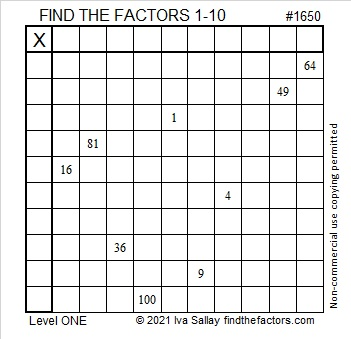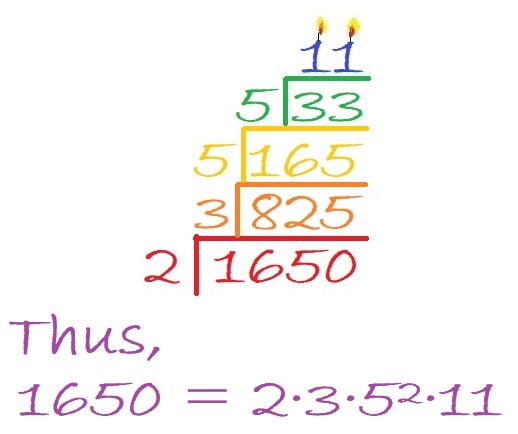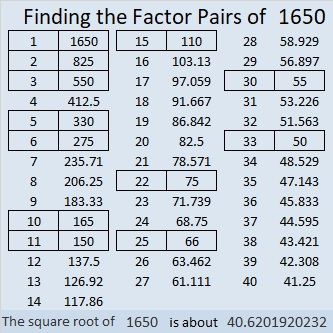# 1650 Wrinkles in the Multiplication Table

Contents

### Today’s Puzzle:

Are you familiar with the book A Wrinkle in Time? Kat of The Lily Cafe’s blog loves books and recently compared Meg in that book to her six-year-old son. She wrote a post titled Am I Raising a Meg? Her six-year-old LOVES math and is very much interested in multiplication and division. When Mom thought he was playing a game on her phone, he was actually playing with the calculator app! I felt so happy inside as I read that!

I wonder if they have discovered the storybooks in the Math Book Magic blog. Such books could combine Mom’s love for reading with her son’s love of math.

Someday her son might like to solve a “wrinkled” multiplication table puzzle like this one that has only nine clues.

Write all the numbers 1 to 10 in both the first column and the top row so that those numbers and the given clues become a multiplication table.### Factor Cake for 1650:

This is my 1650th post.
1650 is divisible by 2 and by 5 because it ends with a 0.
1650 is divisible by 3 because 1 + 6 + 5 + 0 = 12, a number divisible by 3.
1650 is divisible by 11 because 1 – 6 + 5 – 0 = 0, a number divisible by 11.

I think we can make a lovely factor cake for 1650:### Factors of 1650:

• 1650 is a composite number.
• Prime factorization: 1650 = 2 × 3 × 5 × 5 × 11, which can be written 1650 = 2 × 3 × 5² × 11.
• 1650 has at least one exponent greater than 1 in its prime factorization so √1650 can be simplified. Taking the factor pair from the factor pair table below with the largest square number factor, we get √1650 = (√25)(√66) = 5√66.
• The exponents in the prime factorization are 1, 1, 2, and 1. Adding one to each exponent and multiplying we get (1 + 1)(1 + 1)(2 + 1)(1 + 1) = 2 × 2 × 3 × 2 = 24. Therefore 1650 has exactly 24 factors.
• The factors of 1650 are outlined with their factor pair partners in the graphic below.### More About the Number 1650:

1650 is the hypotenuse of TWO Pythagorean triples:
462-1584-1650, which is (7-24-25) times 66, and
990-1320-1650, which is (3-4-5) times 330.

This site uses Akismet to reduce spam. Learn how your comment data is processed.